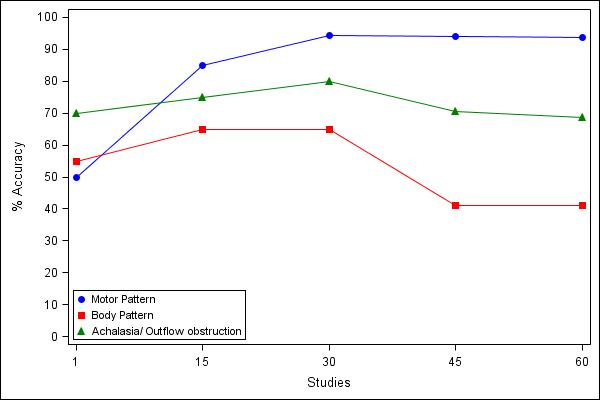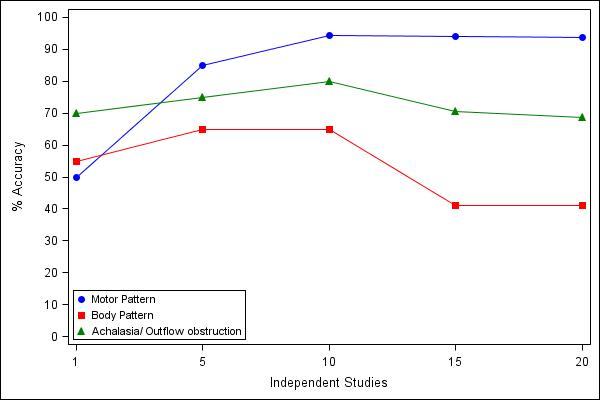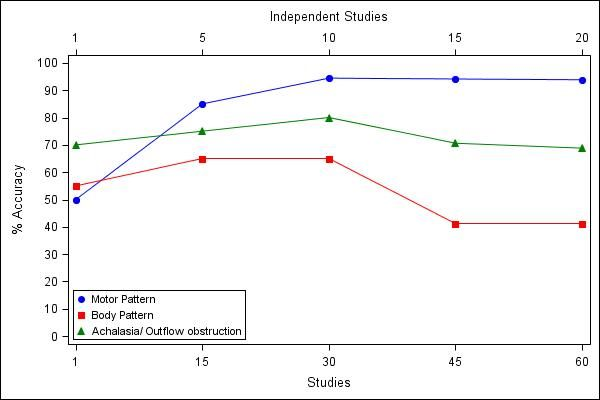## X axis labeling with two labels in same graph

Hi all,

I am looking for help in creating graphs with two sets of labeling for X-axis. I am attaching my excel sheet, SAS code and the graphs  I had generated. Basicially I want two X-axis labeling (Independent Studies and Studies) in the same graph.

Thanks for all the help,

Satish

``````ods listing close;
ods graphics / reset width=600px height=400px imagename='Fig 1' imagefmt=jpeg;
ods html file='Surg.html' path='E:\HRM\Revised\Revised18_New' style=journal;

/*ods html file='ap.html' path='C:\' style=journal; */
proc sgplot data=graphs;
scatter X=Studies Y=MP /  markerattrs=(color=blue symbol=CircleFilled size=8) name="scat" legendlabel="Motor Pattern";
series X=Studies Y=MP /  lineattrs=(color=blue pattern=1) ;
scatter X=Studies Y=BP /  markerattrs=(color=red symbol=SquareFilled size=8) name="scat1" legendlabel="Body Pattern";
series X=Studies Y=BP /  lineattrs=(color=red pattern=1) ;
scatter X=Studies Y=OutOb /  markerattrs=(color=green symbol=TriangleFilled size=8) name="scat2" legendlabel="Achalasia/ Outflow obstruction";
series X=Studies Y=OutOb /  lineattrs=(color=green pattern=1) ;

XAXIS TYPE = DISCRETE ;
YAXIS LABEL = '% Accuracy'    VALUES = (0 TO 100 BY 10);
keylegend "scat" "scat1" "scat2"  "scat4"  / position=bottomleft
across=1 location=inside ;
run;
ods html close;
ods listing;

ods listing close;
ods graphics / reset width=600px height=400px imagename='Fig 1_New' imagefmt=jpeg;
ods html file='Surg.html' path='E:\HRM\Revised\Revised18_New' style=journal;

/*ods html file='ap.html' path='C:\' style=journal; */
proc sgplot data=graphs;
scatter  X=Independent_Studies Y=MP /  markerattrs=(color=blue symbol=CircleFilled size=8) name="scat" legendlabel="Motor Pattern";
series  X=Independent_Studies Y=MP /  lineattrs=(color=blue pattern=1) ;
scatter  X=Independent_Studies Y=BP /  markerattrs=(color=red symbol=SquareFilled size=8) name="scat1" legendlabel="Body Pattern";
series  X=Independent_Studies Y=BP /  lineattrs=(color=red pattern=1) ;
scatter  X=Independent_Studies Y=OutOb /  markerattrs=(color=green symbol=TriangleFilled size=8) name="scat2" legendlabel="Achalasia/ Outflow obstruction";
series X=Independent_Studies Y=OutOb /  lineattrs=(color=green pattern=1) ;

XAXIS TYPE = DISCRETE ;
YAXIS LABEL = '% Accuracy'    VALUES = (0 TO 100 BY 10);
keylegend "scat" "scat1" "scat2"  "scat4"  / position=bottomleft
across=1 location=inside ;
run;
ods html close;
ods listing;
``````1 ACCEPTED SOLUTION

Accepted Solutions

## Re: X axis labeling with two labels in same graph

In this case try this, similar but not exactly what you posted.

I think what you want can be done with GTL but I never tried it.

``````ods listing close;
ods graphics / reset width=600px height=400px imagename="Fig 1" imagefmt=jpeg;
ods html file='Surg.html' path='E:\HRM\Revised\Revised18_New' style=journal;

proc sgplot data=graphs;
scatter  X=Independent_Studies Y=MP      / markerattrs=(color=blue symbol=CircleFilled size=8) legendlabel="Motor Pattern" x2axis;
scatter  X=Studies Y=MP      / markerattrs=(color=blue symbol=CircleFilled size=8) name="scat" legendlabel="Motor Pattern";
series   X=Studies Y=MP      / lineattrs=(color=blue pattern=1) ;
scatter  X=Studies Y=BP      / markerattrs=(color=red symbol=SquareFilled size=8) name="scat1" legendlabel="Body Pattern";
series   X=Studies Y=BP      / lineattrs=(color=red pattern=1) ;
scatter  X=Studies Y=OutOb   / markerattrs=(color=green symbol=TriangleFilled size=8) name="scat2" legendlabel="Achalasia/ Outflow obstruction";
series   X=Studies Y=OutOb   / lineattrs=(color=green pattern=1) ;
XAXIS TYPE = DISCRETE label="Studies";
X2AXIS TYPE = DISCRETE label="Independent Studies";
YAXIS LABEL = '% Accuracy' VALUES = (0 TO 100 BY 10);
keylegend "scat" "scat1" "scat2" / position=bottomleft across=1 location=inside ;
run;
ods html close;
ods listing; ``````________________________

- Cheers -

9 REPLIES 9

## Re: X axis labeling with two labels in same graph

Hi,

put your code in a macro block and parameterize it, like this:

``````data graphs;
infile cards expandtabs truncover;
input Independent_Studies Studies MP MP_Low MP_High BP BP_Low BP_High OutOb Outob_Low OutOb_High Mx Mx_low Mx_High MxEGJ MxEGJ_low MxEGJ_High Fundo Fundo_Low Fundo_High ;
cards;
1 1 50 45 50 55 50 60 70 65 75 80 75 85 68.4 63.2 73.7 66.7 61.1 72.2
5 15 85 80 90 65 60 82.9 75 56.7 80 55 50 60 90 71.9 95 50 39.5 60.5
10 30 94.4 74.7 100 65 46.7 70 80 54.2 85 65 60 70 80 54.2 85 35.3 13.4 59.3
15 45 94.1 73.2 100 41.2 18.1 47.1 70.6 48.9 85.9 76.5 70.6 82.4 66.7 39.8 87.6 42.9 29.3 72.8
20 60 93.8 77.6 100 41.2 18.1 47.1 68.8 52.5 75 81.3 75 87.5 73.3 52.1 85.9 75 52.3 81.2
;
run;

%MACRO doGraph(var=,xlabel=);
ods listing close;
ods graphics / reset width=600px height=400px imagename="Fig 1 &xlabel." imagefmt=jpeg;
ods html file='Surg.html' path='E:\HRM\Revised\Revised18_New' style=journal;

proc sgplot data=graphs;
scatter X=Independent_Studies Y=MP / markerattrs=(color=blue symbol=CircleFilled size=8) name="scat" legendlabel="Motor Pattern";
series X=Independent_Studies Y=MP / lineattrs=(color=blue pattern=1) ;
scatter X=Independent_Studies Y=BP / markerattrs=(color=red symbol=SquareFilled size=8) name="scat1" legendlabel="Body Pattern";
series X=Independent_Studies Y=BP / lineattrs=(color=red pattern=1) ;
scatter X=Independent_Studies Y=OutOb / markerattrs=(color=green symbol=TriangleFilled size=8) name="scat2" legendlabel="Achalasia/ Outflow obstruction";
series X=Independent_Studies Y=OutOb / lineattrs=(color=green pattern=1) ;
XAXIS TYPE = DISCRETE label="&xlabel.";
YAXIS LABEL = '% Accuracy' VALUES = (0 TO 100 BY 10);
keylegend "scat" "scat1" "scat2" "scat4" / position=bottomleft across=1 location=inside ;
run;

ods html close;
ods listing;
%MEND doGraph;

%doGraph(var=Studies,xlabel=Studies);
%doGraph(var=Independent_Studies,xlabel=Independent Studies);``````

Cheers,

Oligolas

________________________

- Cheers -

## Re: X axis labeling with two labels in same graph

Thank you Oligolas. I ran your code. I did not get any errors, but it just gives me only one x-axis labeling (independent studies) and not for both. Can you help? I have SAS 9.4.

Thanks a lot again!

Satish

## Re: X axis labeling with two labels in same graph

Hi I am not sure to understand?

Do you mean in the HTML? do you want both jpg in the same HTML?

like this?

``````%MACRO doGraph(var=,xlabel=);
ods listing close;
ods graphics / reset width=600px height=400px imagename="Fig 1 &xlabel." imagefmt=jpeg;

proc sgplot data=graphs;
scatter X=Independent_Studies Y=MP / markerattrs=(color=blue symbol=CircleFilled size=8) name="scat" legendlabel="Motor Pattern";
series X=Independent_Studies Y=MP / lineattrs=(color=blue pattern=1) ;
scatter X=Independent_Studies Y=BP / markerattrs=(color=red symbol=SquareFilled size=8) name="scat1" legendlabel="Body Pattern";
series X=Independent_Studies Y=BP / lineattrs=(color=red pattern=1) ;
scatter X=Independent_Studies Y=OutOb / markerattrs=(color=green symbol=TriangleFilled size=8) name="scat2" legendlabel="Achalasia/ Outflow obstruction";
series X=Independent_Studies Y=OutOb / lineattrs=(color=green pattern=1) ;
XAXIS TYPE = DISCRETE label="&xlabel.";
YAXIS LABEL = '% Accuracy' VALUES = (0 TO 100 BY 10);
keylegend "scat" "scat1" "scat2" "scat4" / position=bottomleft across=1 location=inside ;
run;
ods listing;
%MEND doGraph;

ods html file='Surg.html' path='E:\HRM\Revised\Revised18_New' style=journal;
%doGraph(var=Studies,xlabel=Studies);
%doGraph(var=Independent_Studies,xlabel=Independent Studies);
ods html close; ``````
________________________

- Cheers -

## Re: X axis labeling with two labels in same graph

Thank you for the reply. No that is not I was looking for. Can you see my PDf attacment? The example I have on PDF is what exactly I am looking for. Is this possible?

## Re: X axis labeling with two labels in same graph

Hi,

ok I understand,

with the x2axis statement it is possible to display 2 axis on one graph...

BUT:

when independent_study has the value of 15 you have and MP value of 85

when study has the value of 15 you have and MP value of 94.1

The figure you draw is unfortunately not correct, rethink about how you want to display the data.

Cheers

________________________

- Cheers -

## Re: X axis labeling with two labels in same graph

Yes, the figure is correct. That is how I want. For x-axis, the value of independent_studies 5 is the same as studies 15. So the data is exactly same but I need two different x-axis exactly how I have in the PDF. will it be possible? I hope I clarified the point.

5 independent studies = 15 studies.

## Re: X axis labeling with two labels in same graph

Independent_study 1 = Study 1

Independent_study 5 = Study 15

Independent_study 10 = Study 30

Independent_study 15 = Study 45

Independent_study 20 = Study 30

## Re: X axis labeling with two labels in same graph

In this case try this, similar but not exactly what you posted.

I think what you want can be done with GTL but I never tried it.

``````ods listing close;
ods graphics / reset width=600px height=400px imagename="Fig 1" imagefmt=jpeg;
ods html file='Surg.html' path='E:\HRM\Revised\Revised18_New' style=journal;

proc sgplot data=graphs;
scatter  X=Independent_Studies Y=MP      / markerattrs=(color=blue symbol=CircleFilled size=8) legendlabel="Motor Pattern" x2axis;
scatter  X=Studies Y=MP      / markerattrs=(color=blue symbol=CircleFilled size=8) name="scat" legendlabel="Motor Pattern";
series   X=Studies Y=MP      / lineattrs=(color=blue pattern=1) ;
scatter  X=Studies Y=BP      / markerattrs=(color=red symbol=SquareFilled size=8) name="scat1" legendlabel="Body Pattern";
series   X=Studies Y=BP      / lineattrs=(color=red pattern=1) ;
scatter  X=Studies Y=OutOb   / markerattrs=(color=green symbol=TriangleFilled size=8) name="scat2" legendlabel="Achalasia/ Outflow obstruction";
series   X=Studies Y=OutOb   / lineattrs=(color=green pattern=1) ;
XAXIS TYPE = DISCRETE label="Studies";
X2AXIS TYPE = DISCRETE label="Independent Studies";
YAXIS LABEL = '% Accuracy' VALUES = (0 TO 100 BY 10);
keylegend "scat" "scat1" "scat2" / position=bottomleft across=1 location=inside ;
run;
ods html close;
ods listing; ``````________________________

- Cheers -

## Re: X axis labeling with two labels in same graph

Thanks, this works!

Satish

Discussion stats
• 9 replies
• 2415 views
• 1 like
• 2 in conversation